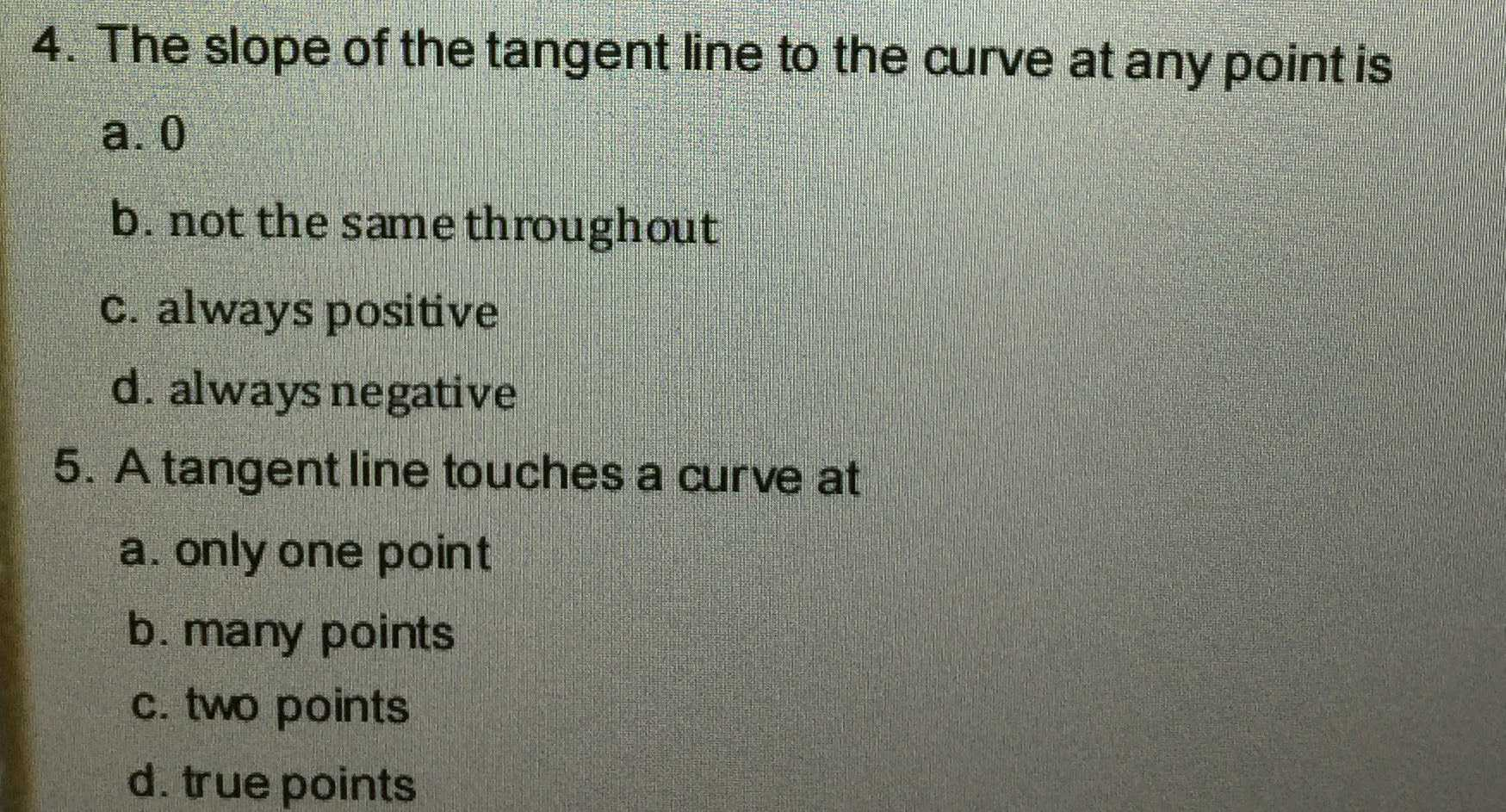### ¿Todavía tienes preguntas de matemáticas?

Pregunte a nuestros tutores expertos
Algebra
Pregunta1. The slope of the tangent line to the curve at any point is a. $$0$$ b. not the same throughout c. always positive d. always negative 5. A tangent line touches a curve at a. only one point b. many points c. two points d. true points

a. $$0$$

b. not the same throughout

c. always positive

d. always negative

2. A tangent line touches a curve at

a. only one point

b. many points

c. two points

d. true points